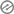# Physical Chemistry MCQ on Thermodynamics

## Physical Chemistry MCQ on Thermodynamics

1. Foe which thermodynamic process the change in enthalpy is zero?

(a) isothermal

(b) isochoric

(c) isobaric

`Answer: (d) adiabatic`

2. What is the value of gamma for gas if the pressure of the gas is proportional to the T³ for adiabatic process ?

(a) 2

(b) 3/2

(c) 4/3

(d) 5/3

`Answer: (b) 3/2`

3. The coefficient performance of a refrigerator is 5. Calculate the heat emitted to the surrounding if the temperature inside system is -20⁰c.(a) 11oC

(b) 41oC

(c) 21oC

(d) 31oC

`Answer: (d) 31oC`

4. According to Kirchhoff equation Which of the following factor affects the heat of reaction

(a) molecularity

(b) temperature

(c) pressure

(d) volume`Answer: (b) temperature`

5. Chemical dissociation is

(a) exothermic

(b) reversible(c) endothermic

(d) reversible and endothermic

`Answer: (d) reversible and endothermic`

6. The largest unit of energy is

(a) electron volt

(b) joule

(c) calorie

(d) erg

`Answer: (c) calorie`

7.  Aunique characteristic feature of perfect black body is

(a) a good absorber only(c) a good absorber and a good radiator

(d) neither a radiator nor an absorber

`Answer: (c) a good absorber and a good radiator`

8. The thermodynamic process where heat cannot be exchanged within system and surrounding is

(a) isothermal

(c) isobaric

(d) isotropic

`Answer: (b) adiabatic`

9. The law that was formulated by Nernst is

(a) First law of thermodynamics

(b) Second law of thermodynamics

(c) Third law of thermodynamics(d) None of the above

`Answer: (c) Third law of thermodynamics`